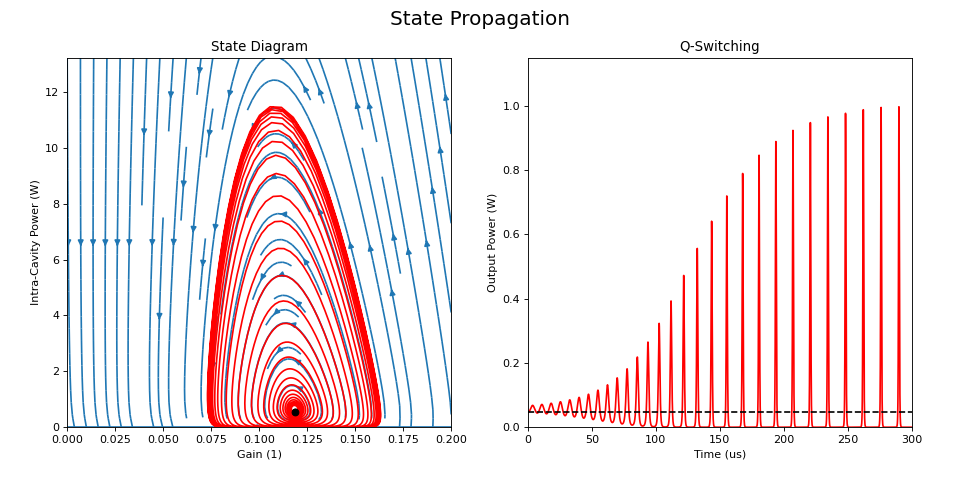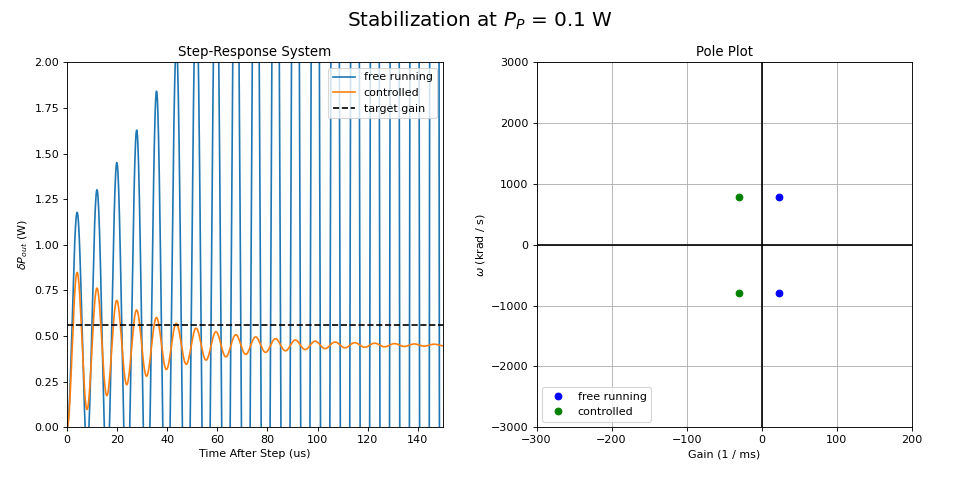# Mode-Locked Laser¶

A mode-locked laser is a type of laser that operates in pulsed mode. This is achieved, e.g., by placing a saturable absorber inside the laser cavity. The saturable absorber has high losses for low light intensity but low losses for high intensity. This forces the laser to concentrate its light in short (and therefore intense) pulses.

However, the saturable absorber also leads to stability issues: When the pulse energy increases from its steady-state value, the saturable losses decrease - and vice versa. This tends to amplify deviations from the steady state and leads to so called Q-switched mode locking if not properly controlled. In Q-switched mode locking the laser emits bunches of pulses instead of a continuous stream of equally strong pulses.

Typically, Q-switched mode locking is avoided by proper design of the laser. Here, we don’t do that. Instead, we design a state-feedback controller that stabilizes the laser by acting on its pump power.

The mode-locked laser is governed by the following differential equations:

\begin{split}\begin{align*} \dot P &= \frac{g - l - q_P(E_P)}{T_R} \cdot P \\ \dot g &= \frac{\eta_P P_P}{E_{sat,L}} - \frac{g}{\tau_L} - \frac{P \cdot g}{E_{sat,L}} \\ q_P(S = E_P / E_{sat,A}) &= \frac{\Delta R}{S} \cdot \left(1 - e^{-S} \right) \end{align*}\end{split}

where $$P$$ the power inside the laser cavity, $$g$$ the gain provided by the gain medium, $$l$$ and $$q_P(E_P)$$ the linear and non-linear losses, respectively, $$E_P = P \cdot T_R$$ the pulse energy, and $$T_R$$ the time it takes the pulse to travel around the cavity once. $$E_{sat,L}$$ and $$E_{sat,A}$$ are the saturation energies of the gain medium and the saturable absorber, respectively, and $$\tau_L$$ the relaxation time of the gain.

The examples package includes the module ‘laser’ that provides a class to simulate such a laser. The class also includes a method ‘approximateLTI’ that returns the linear approximation around the steady state, i.e., a CT_LTI_System.

## Q-Switching Instability¶

First, let’s have a look at this Q-switching instability. We instantiate the NdYVO4 laser class defined in the examples package and choose a low pump power to assure that it Q-switches (0.1 Watts is appropriate). Then, we solve the differential equations to obtain $$P(t)$$ and $$g(t)$$:

from tanuna.examples.laser import NdYVO4Laser
import numpy as np

Ppump = 0.1
NdYVO4 = NdYVO4Laser(Ppump)

# ODE solving
# =============================================================================

t = np.arange(6000) * NdYVO4.TR * 5
P, g = np.zeros(t.shape), np.zeros(t.shape)

for i in range(len(t)):
P[i], g[i] = NdYVO4.integrate(t[i])



The streamplot below shows that the laser’s state spirals away from the (unstable) steady state towards a limit cycle. The energy of the mode-locked pulses (and therefore $$P_{out}$$) is pulsating. This is what we will elliminate in the next section.## Control¶

By linear approximation around the (unstable) steady-state, we create a second order LTI. This system is also modified so that it not only provides the laser power as output but the internal state as well:

\begin{split}\begin{align*} \dot{\vec{x}} &= A \vec{x} + B u \\ \vec{y} &= \left[ \begin{array}{ccc} 0 & T_{oc} \\ 1 & 0 \\ 0 & 1 \end{array} \right] \vec{x} \end{align*}\end{split}

where $$\vec{x} = \left[ \delta \dot{P} / \omega_0, \delta P \right]^T$$ is the (transformed!) state and $$u = \delta P_P$$ the deviation from the deviation from the (design-) pump power.

from tanuna.examples.laser import NdYVO4Laser
import numpy as np
import tanuna as dyn

# Setup laser
# =============================================================================

Ppump = 0.1
NdYVO4 = NdYVO4Laser(Ppump)

# Linearized
# =============================================================================

M, system = NdYVO4.approximateLTI()
A, B, C, D = system.ABCD
Toc = NdYVO4.Toc
C = np.matrix([[0, Toc],
[1, 0],
[0, 1]])
D = np.matrix(np.zeros((3, 1)))
system = dyn.CT_LTI_System(A, B, C, D)


Next, we add (state-) feedback to obtain the controlled system:Block diagram of laser with state feedback.

$$r$$ is the control input, $$k_r$$ a constant, and $$K = \left[ 0, k_1, k_2 \right]$$ the feedback matrix.

We can now choose $$K$$ in such a way that the stabilized systems has poles where we want them. It can be shown that to obtain poles at:

$p_{1, 2} = -\gamma \omega_0 \pm \sqrt{\nu} |\omega_0|$

we have to choose

$K = \left[ 0 , 2 (\gamma - \zeta) / \rho , (\gamma^2 - \nu - 1) / \rho \right]$

where $$\zeta$$ is the damping ratio of the free-running system. Further, we choose $$k_r = \gamma^2 - \nu$$ to obtain the same DC-gain as in the uncontrolled system. If the system is known perfectly and if the feedback is implemented exactly as calculated, the dynamics of the controlled system will be exactly as intended. In reality, neither is true. Therefore, we assume errors in the knowledge of $$P_P$$ (factor 1.5) and the calibration of the feedback, i.e., in $$k_r$$, $$k_1$$, and $$k_2$$ (factor of 0.8, each):

# Where we want the poles to be:
gamma = 0.05
nu = -1.
# => poles will be at -gamma * w0 +/- sqrt(nu) * w0 = -0.05 * w0 +/- i * w0

# We assume that the controller is not calibrated perfectly.
#   a) The assumed pump power is factor rPp from real pump power
#   b) The implemented feedback is factors rkr, rk1, rk2 from calculated values
# Note how large we choose the errors!
rPp = 1.5
rkr = 0.8
rk1 = 0.8
rk2 = 0.8

# Calculate and apply feedback:
NdYVO4.PP = rPp * NdYVO4.PP
kr = rkr * (gamma**2 - nu)
k1 = rk1 * 2. * (gamma - NdYVO4.zeta()) / NdYVO4.rho()
k2 = rk2 * (gamma**2 - nu - 1.) / NdYVO4.rho()

stateoutput = np.matrix([[1, 0, 0]])
K = np.matrix([[0, k1, k2]])
L = np.vstack([stateoutput, K])
summing = np.matrix([kr, -1])
stabilized = L * system * summing
stabilized = dyn.feedback(stabilized, Gout=(1,), Gin=(1,))


Now, let’s compare to the free-running system. The figure below shows the step-response and the poles of the free-running and the controlled system. As expected, the relaxation oscillation are damped, resulting in a stable system. The gain is not exactly what we aimed for (green curve is not converging towards the target gain (dashed line). Given the large errors we have assumed this is - however - still a quite acceptable result.